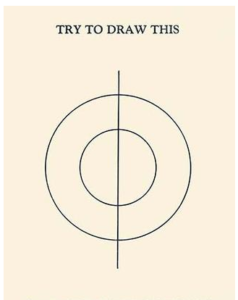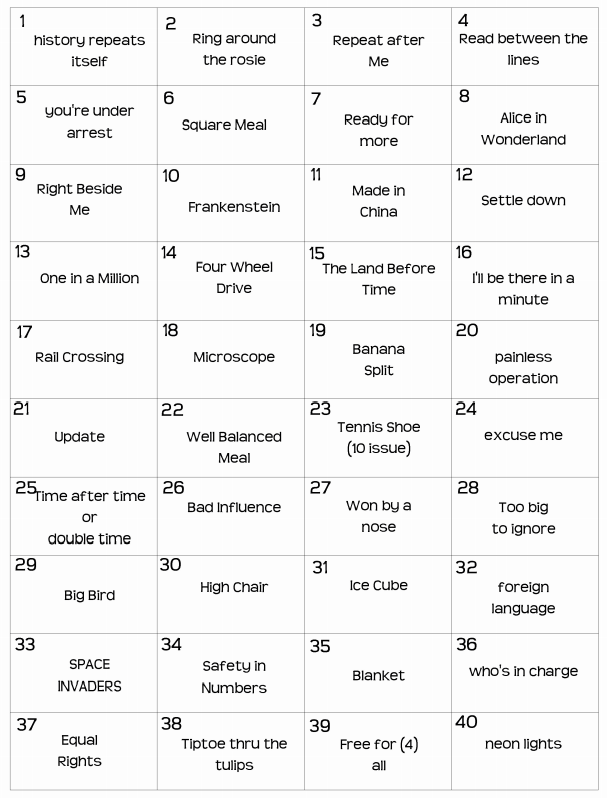Select Page

# Brain Practice

April 2017

## Did you try each several times?

Here are the answers to Brain Practice,  April 2017

#### Draw without crossing the line

No set starting point for this – did you manage it?#### Fill in the Blanks

P  R  O  N  U  N  C  I  A  T  I  O  N

#### English Words

We found only two! Did you find any others?

NEIGH,  HINGE

#### Can you Solve These?Who Gets what?

Using the ‘guess and check’ method, it goes like this:

1. Try \$24 and \$1 – the first part is 24 times the second part. Not quite enough!
2. Now halve the \$1 to give two lots of 50 cents and add one lot to John’s \$24.00.
3. Now John’s part is \$24.50 and Bill’s part is 50 cents.
4. John has 49 times as much as Bill.  Poor Bill He didn’t do very well out of that!

(Dividing a number by a half is the same as multiplying it by 2. that means that dividing \$24.50 by 50 cents (half a dollar), we get the result 49.

Mathematicians, of course, will set up a pair of simultaneous equations and solve them that way!